# Areas of Shapes

GCSEKS3Level 4-5Level 6-7AQACambridge iGCSEEdexcelEdexcel iGCSEOCRWJEC

## Areas of Shapes

The area of a 2D shape is the amount of space it takes up in $2$ dimensions, and its units are always squared, e.g

$\text{cm}^2,\hspace{1mm}\text{m}^2$

You need to know the formulas to calculate the areas of some common shapes and be able to rearrange them. Revising rearranging formulae will help with this topic.

Level 4-5GCSEKS3AQAEdexcelOCRWJECCambridge iGCSEEdexcel iGCSE

## Area of Rectangle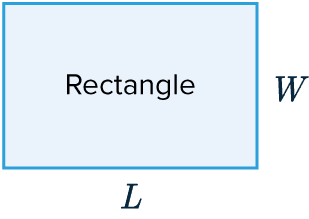The area of a rectangle is length $\times$ width

$\text{Area} = L \times W$

Level 4-5GCSEKS3AQAEdexcelOCRWJECCambridge iGCSEEdexcel iGCSE

## Area of a Parallelogram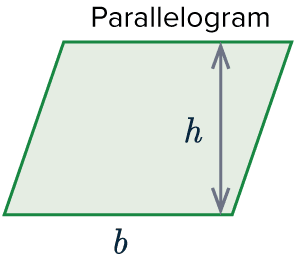The area of a parallelogram is base $\times$ vertical height

$\text{Area} = b\times h$

Level 4-5GCSEKS3AQAEdexcelOCRWJECCambridge iGCSEEdexcel iGCSE

## Area of a Trapezium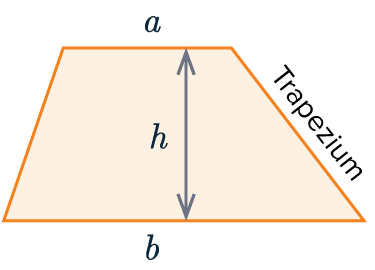The formula to calculate the area of a trapezium is:

$\text{Area} = \dfrac{1}{2}(a+b)h$

where $a$ and $b$ are the lengths of the parallel sides and $h$ is the vertical height.

Level 4-5GCSEKS3AQAEdexcelOCRWJECCambridge iGCSEEdexcel iGCSE
Level 6-7GCSEAQAEdexcelOCRWJECCambridge iGCSEEdexcel iGCSE

## Area of a Triangle 1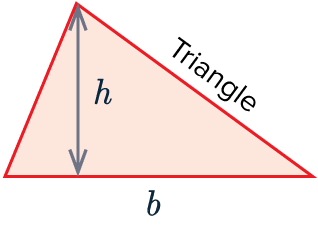The equation to calculate the area of a triangle is:

$\text{Area} = \dfrac{1}{2} \times b \times h$

Where $b$ is the base width of the triangle and $h$ is the vertical height.

Level 4-5GCSEKS3AQAEdexcelOCRWJECCambridge iGCSEEdexcel iGCSE
Level 6-7GCSEAQAEdexcelOCRWJECCambridge iGCSEEdexcel iGCSE

## Area of a Triangle 2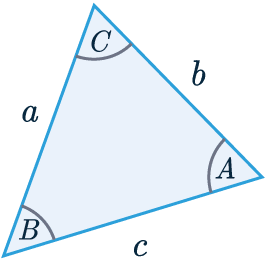Another way to calculate the area of a triangle is as follows:

$\dfrac{1}{2} \times a \times b \times \sin(C)$

Where $a$ and $b$ are side lengths and $C$ is the angle between the side lengths.

Level 6-7GCSEAQAEdexcelOCRWJECCambridge iGCSEEdexcel iGCSE

## Example 1: Finding the Area of a Trapezium

The shape is a trapezium with a perpendicular height of $4$mm.

Calculate the area of the trapezium.

[2 marks]Formula: $\text{Area}=\frac{1}{2}(a+b)h$,

where $a = 8,\hspace{1mm}b = 12.5,$ and $h = 4$.

\begin{aligned}\text{Area } &= \dfrac{1}{2}(8+12.5) \times 4 \\ &=\dfrac{1}{2} \times 20.5 \times 4 \\ &= 41\text{ mm}^2 \end{aligned}

Level 4-5GCSEKS3AQAEdexcelOCRWJECCambridge iGCSEEdexcel iGCSE

## Example 2: Area of a Triangle

The triangle has a base of $6$cm and an area equal to $24\text{ cm}^2$.

Calculate its perpendicular height.

[2 marks]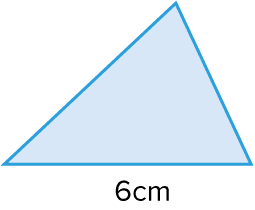Formula: $\text{Area} = \dfrac{1}{2}\times b \times h$

So we can add the numbers we know into the equation and solve for $h$:

$24= \dfrac{1}{2}\times 6 \times h$

$24= 3 \times h$

$\dfrac{24}{3}= h$

$h = 8\text{cm}$

Level 4-5GCSEKS3AQAEdexcelOCRWJECCambridge iGCSEEdexcel iGCSE

## Areas of Shapes Example Questions

The formula for the area is $\frac{1}{2} \times b \times h$, where $b = 11.5 \text{ and } h = 12$. So, we get:

$\text{Area } = \dfrac{1}{2} \times 11.5 \times 12 = 69\text{cm}^2$Gold Standard Education

To work out the area, we will need to find the perpendicular height by forming a right-angled triangle,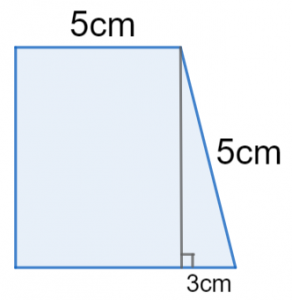The hypotenuse of the right-angled triangle is 5cm and the base is 3cm (8cm-5cm=3cm), so we get the perpendicular height of the trapezium as,

$\text{Perpendicular height} = \sqrt{5^2 - 3^2} = \sqrt{16} = 4\text{cm}$

Now we know the perpendicular height, we can calculate the area.

$\text{Area} = \dfrac{1}{2}(a + b)h = \dfrac{1}{2}(5 + 8) \times 4 = 26\text{cm}^2$Gold Standard Education

Area of a parallelogram is given by the formula,

$\text{Area}=\text{base}\times\text{height}$.

Therefore,

$\text{Area}=8 \times 15 =120\text{cm}^2$Gold Standard Education

As we need to find a missing side-length rather than the area, we’re going to have to set up an equation and rearrange it to find $x$. The formula for the area we’ll need here is

$\dfrac{1}{2}ab \sin(C)$,

so, our equation is

$\dfrac{1}{2} \times 2.15 \times x \times \sin(26) = 1.47$

Simplifying the left-hand-side, we get

$1.075 \sin (26) \times x = 1.47$

Finally, dividing through by $1.075\sin(26)$, and putting it into a calculator, we get

$x = \dfrac{1.47}{1.075\sin(26)} = 3.12\text{m (2 d.p.)}$Gold Standard Education

## Areas of Shapes Worksheet and Example Questions

### (NEW) Areas of Shapes Exam Style Questions - MME

Level 4-5Level 6-7GCSENewOfficial MME

## Areas of Shapes Drill Questions

### Area and Volume - Drill Questions

Level 4-5GCSEProduct